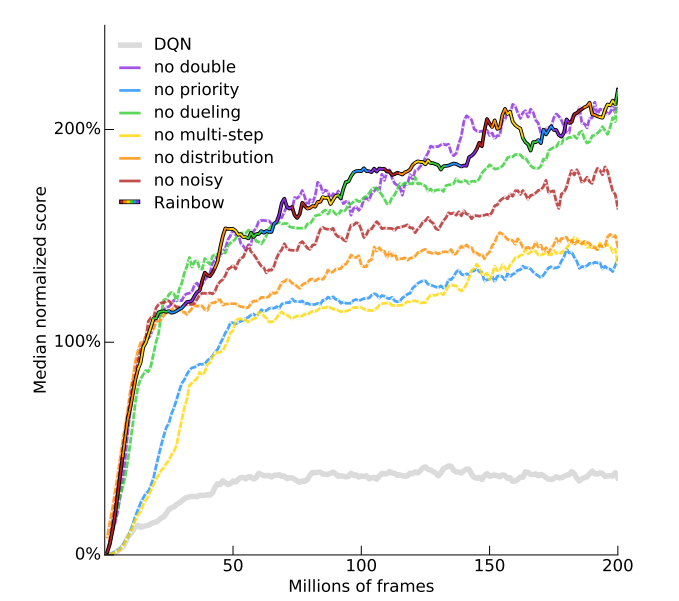# 强化学习的学习之路（二十八）_2021-01-28：Rainbow

##### Rainbow

Rainbow一个集多长所长的模型，来自论文 Rainbow: Combining Improvements in Deep Reinforcement Learning，他主要包括我们前面提到的一下几个改进，我们这里稍微再回顾一下：

###### Double Q-Learning

Double Q-Learning 构建了两个结构相同但是参数数值不同的模型：Behavior Net-work 和 Target Network。在模型更新时，首先由 Behavior Network 选出 t + 1 t+1 时刻的最优行动，然后由 Target Network 得到估计 t + 1 t+1 时刻最优行动的目标价值估计。通过将这两个步骤解耦，我们可以减少 Q-Learning 方法对价值过高估计带来的影响，其中的核心公式为
L ( θ ) = ( r t + 1 + γ q θ − ( s t + 1 , argmax ⁡ a ′ q θ ( s t + 1 , a ′ ) ) − q θ ( s t , a t ) ) 2 L(\theta)=\left(r_{t+1}+\gamma q_{\theta^{-}}\left(s_{t+1}, \operatorname{argmax}_{a^{\prime}} q_{\theta}\left(s_{t+1}, a^{\prime}\right)\right)-q_{\theta}\left(s_{t}, a_{t}\right)\right)^{2}

###### Prioritized Replay Buffer

Prioritized Replay Buffer 通过对 Replay Buffer 中不同的样本赋子不同的权重，使得 模型有更高的概率训练对自己有更多提升的样本上, 同时以较低的概率训练对自己提升有限的样本。样本出现的权重和样本采样时的 TD-Error有关，样本的学习率和更新权重还可以根据参数进行调整。

###### Dueling Networks

Dueling Networks 将状态行动价值模型 q ( s , a ) q(s, a) 分解成状态价值 v ( s ) v(s) 和价值优势 A ( s , a ) A(s, a) 两个部分，分解后的两部分具有明确的含义，而这样的分解也同时降低了训练的难度，其中的核心公式为

q ( s t , a t ) = v ( s t ) + ( A ( s t , a t ) − 1 ∣ A ∣ ∑ a ′ A ( s t , a t ′ ) ) q\left(s_{t}, a_{t}\right)=v\left(s_{t}\right)+\left(A\left(s_{t}, a_{t}\right)-\frac{1}{|A|} \sum_{a^{\prime}} A\left(s_{t}, a_{t}^{\prime}\right)\right)

###### Multi-step Learning

q ′ ( s t , a t ) = r t + 1 + γ r t + 2 + ⋯ + γ n − 1 r t + n + max ⁡ a γ n q ( s t + n + 1 , a ) q^{\prime}\left(s_{t}, \boldsymbol{a}_{t}\right)=r_{t+1}+\gamma \boldsymbol{r}_{t+2}+\cdots+\gamma^{n-1} r_{t+n}+\max _{a} \gamma^{n} q\left(s_{t+n+1}, \boldsymbol{a}\right)

###### Distributional Network

DQN 网络只输出了期望形式的价值，而对价值缺少更细致的刻画。Distributional Network 的模型结构可以输出价值的分布形式。我们可以设定价值模型可能的输出范围 [ V M I N , V M A X ] , \left[V_{\mathrm{MIN}}, V_{\mathrm{MAX}}\right], 并在范围内以直方图的形式表示价值对应的出现概率，这使模型的表现能力有了很大的提升，分布形式模型的表示形式如下所示：
{ z i = V M I N + i Δ z : 0 ⩽ i < N } p i ( x , a ) = e θ i ( x , a ) ∑ j e θ j ( x , a ) \begin{array}{l} \left\{z_{i}=V_{\mathrm{MIN}}+i \Delta z: 0 \leqslant i<N\right\} \\ p_{i}(x, a)=\frac{\mathrm{e}^{\theta_{i}(\boldsymbol{x}, a)}}{\sum_{j} \mathrm{e}^{\theta_{j}(\boldsymbol{x}, \boldsymbol{a})}} \end{array}

###### Noisy Network

L ( θ ) = E ϵ − , ϵ [ E ( s t , a t , r t , s t + 1 ) ∼ D [ r t + γ max ⁡ a ∗ ∈ A Q ( s t + 1 , a ∗ , ϵ − ; θ − , σ − ) − Q ( s t , a t , ϵ ; θ , σ ) ] 2 ] \begin{aligned} L(\theta)=& E_{\epsilon^{-}, \epsilon}\left[E_{\left(s_{t}, a_{t}, r_{t}, s_{t+1}\right) \sim D}\left[r_{t}+\gamma \max _{a * \in A} Q\left(s_{t+1}, a^{*}, \epsilon^{-} ; \theta^{-}, \sigma^{-}\right)\right.\right.\\ &\left.\left.-Q\left(s_{t}, a_{t}, \epsilon ; \theta, \sigma\right)\right]^{2}\right] \end{aligned}

###### 如何结合:

d t ( n ) = ( R t ( n ) + γ t ( n ) z , p θ ˉ ( S t + n , a t + n ∗ ) ) d_{t}^{(n)}=\left(R_{t}^{(n)}+\gamma_{t}^{(n)} z, \quad \boldsymbol{p}_{\bar{\theta}}\left(S_{t+n}, a_{t+n}^{*}\right)\right)

D K L ( Φ z d t ( n ) ∥ d t ) D_{\mathrm{KL}}\left(\Phi_{z} d_{t}^{(n)} \| d_{t}\right)

p t ∝ ( D K L ( Φ z d t ( n ) ∥ d t ) ) ω p_{t} \propto\left(D_{\mathrm{KL}}\left(\Phi_{z} d_{t}^{(n)} \| d_{t}\right)\right)^{\omega}

p θ i ( s , a ) = exp ⁡ ( v η i ( ϕ ) + a ψ i ( ϕ , a ) − a ˉ ψ i ( s ) ) ∑ j exp ⁡ ( v η j ( ϕ ) + a ψ j ( ϕ , a ) − a ˉ ψ j ( s ) ) p_{\theta}^{i}(s, a)=\frac{\exp \left(v_{\eta}^{i}(\phi)+a_{\psi}^{i}(\phi, a)-\bar{a}_{\psi}^{i}(s)\right)}{\sum_{j} \exp \left(v_{\eta}^{j}(\phi)+a_{\psi}^{j}(\phi, a)-\bar{a}_{\psi}^{j}(s)\right)}01-06237
02-2570
02-2625
06-251078
12-2989
10-111627
01-241001
07-172340
02-2675
02-2646
02-2622
02-2683
11-147207
03-25807
05-313081
02-2533
02-2641
©️2020 CSDN 皮肤主题: 大白 设计师:CSDN官方博客